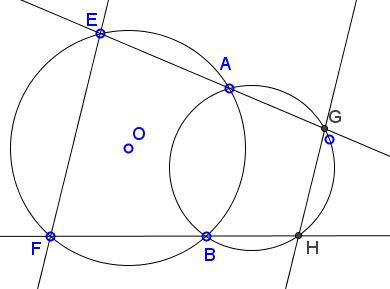# Reim's Similar Coins I

### Problem

Let two circles cross at points $A$ and $B;$ $E$ and $F$ are two points on one of the circles; $EA$ meets the second circle second time at $G,$ $FB$ at $H:$Then $GH\parallel EF.$

### Solution

Chasing angles leads to a simple solution.By the construction, angles $BAE$ ad $BAC$ are supplementary: $\angle BAE+\angle BAG=180^{\circ}.$ On the other hand, quadrilateral $ABHG$ is cyclic, implying $\angle BAG+\angle BHG=180^{\circ}.$ Hence, $\angle BHG=\angle BAE.$ Similarly, since quadrilateral $AEFB$ is cyclic $\angle BAE+\angle BFE=180^{\circ}$ which gives $\angle BHG+\angle BFE=180^{\circ}.$ These I believe are called "consecutive interior angles". Having them add up to $180^{\circ}$ makes the two lines, $GH$ and $EF,$ parallel.

### Converse 1

Given a cyclic quadrilateral $ABFE$ and points $G$ and $H$ on the extensions of $EA$ and $FB,$ respectively. If $GH\parallel EF,$ then quadrilateral $ABHG$ is cyclic.### Converse 2

Let two circles cross at points $A$ and $B;$ $E$ and $F$ are two points on one of the circles; $EF$ meets the second circle second time at $G,$ $GH$ a chord in the second circle such that at $GH\parallel EF.$Then $FH$ passes through $B.$

For, if $FH'$ through $B,$ with $H'$ on the second circle, then $GH\parallel EF\parallel GH',$ implying that $H=H'.$

### Acknowledgment

I confess to not knowing the reason for the theorem designation. There is a companion theorem under the same attribution. I came across the latter in an article by Jean-Louis Ayme where he referred to it as "Le théorème des moniennes semblables de Reim" which both I and google had a difficulty translating. It looks to me like "Reim's similar coins" might be a good fit, but I am not sure.